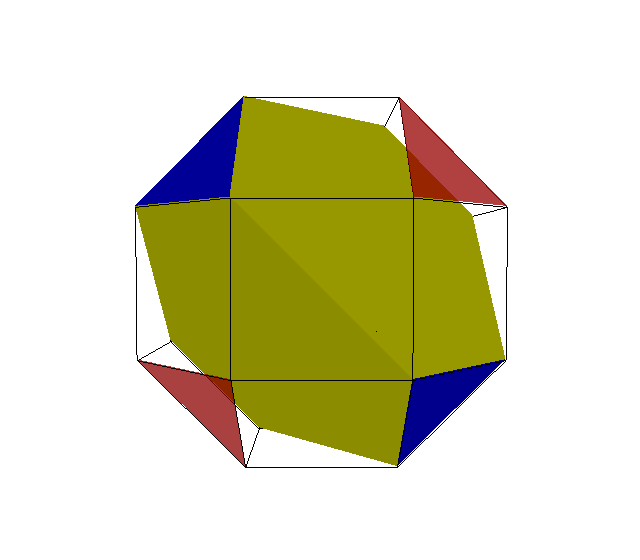Acronym ... Name q3x3o,variation of truncated tetrahedron,triangle-snub small rhombicuboctahedron `` Circumradius sqrt[5+2 sqrt(2)]/2 = 1.398966 General of army (is itself convex) Colonel of regiment (is itself locally convex) Dihedral angles between x3o and q3x:   arccos(-1/3) = 109.471221° between q3x and q3x (at q):   arccos(1/3) = 70.528779° Confer uniform variant: tut   variations: a3b3c   x3v3o   x3q3o   x3u3o   x3w3o   v3x3o   u3x3o   w3x3o   (-x)3x3o

using edge sizes x = 1 and q = sqrt(2) = 1.414214

This polyhedron can be vertex inscribed into sirco. In fact, the latter is just a tegum sum of 2 such in dual position. Conversely it can be derived from sirco by means of a mere alternated faceting, cf. the above picture.

Incidence matrix according to Dynkin symbol

```q3x3o

. . . | 12 | 1  2 | 2 1
------+----+------+----
q . . |  2 | 6  * | 2 0
. x . |  2 | * 12 | 1 1
------+----+------+----
q3x . |  6 | 3  3 | 4 *
. x3o |  3 | 0  3 | * 4
```

```o3/2x3q

.   . . | 12 |  2 1 | 1 2
--------+----+------+----
.   x . |  2 | 12 * | 1 1
.   . q |  2 |  * 6 | 0 2
--------+----+------+----
o3/2x . |  3 | 3  0 | 4 *
.   x3q |  6 | 3  3 | * 4
```